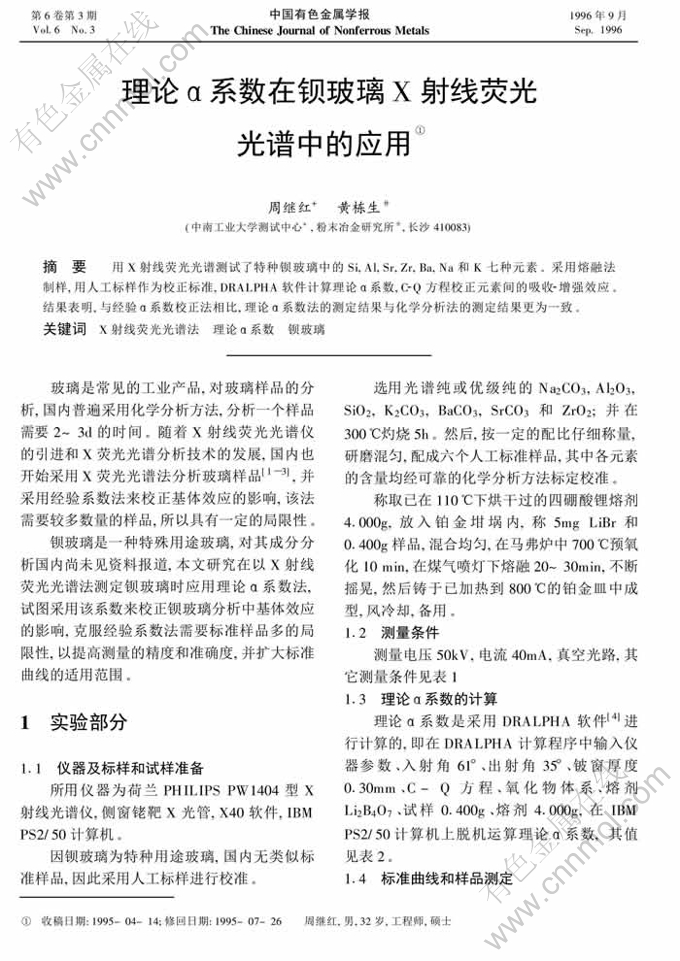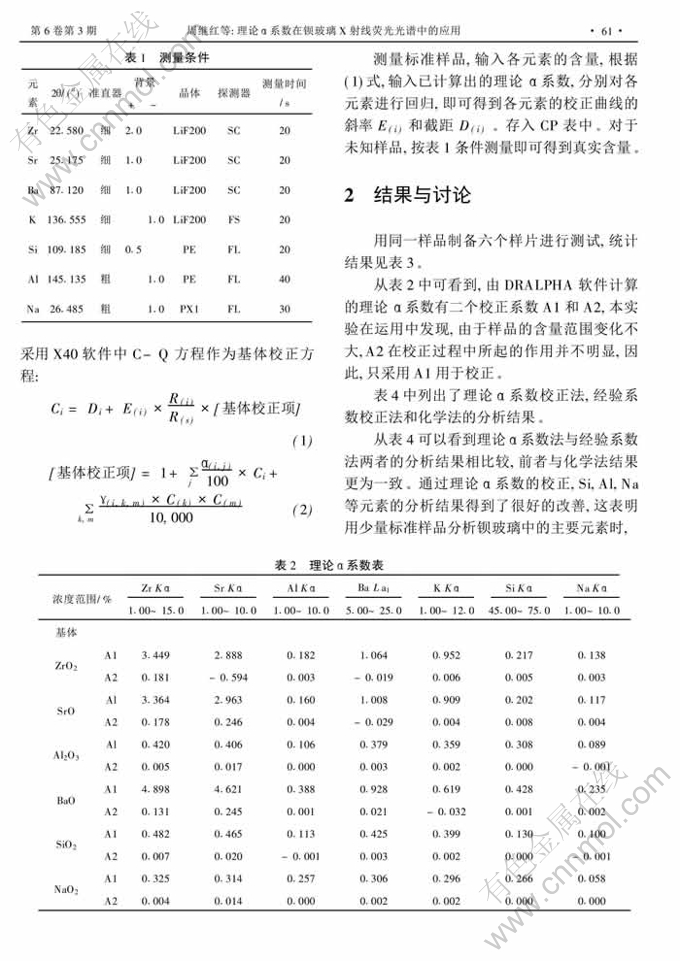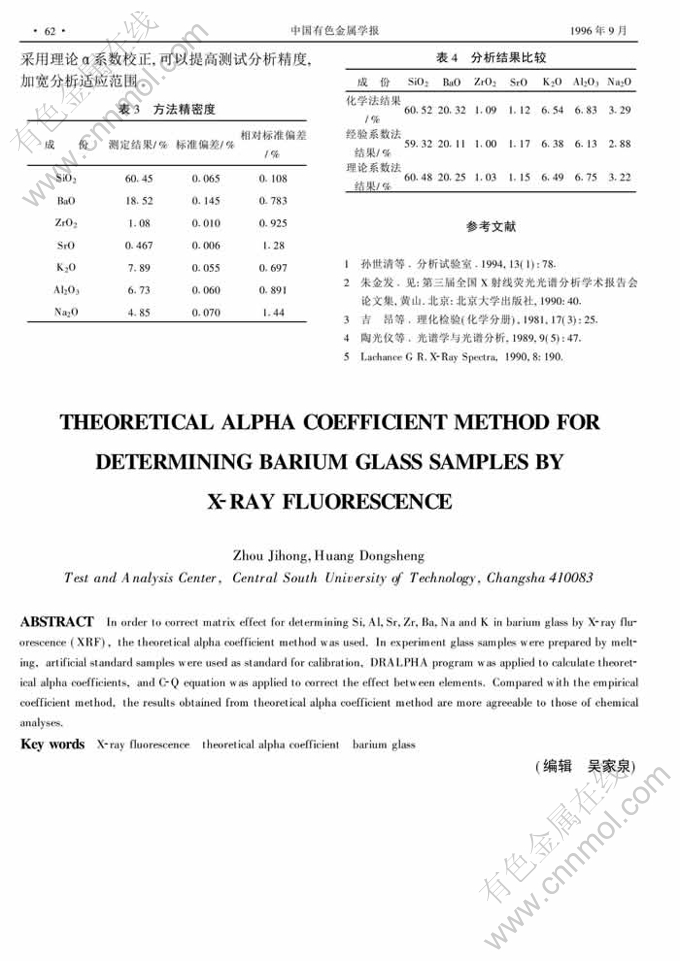## 简介概要

Key words：X-ray fluorescence　theoretical alpha coefficient　barium glass

Abstract: In order to correct matrix effect for determining Si,Al,Sr,Zr,Ba,Na and K in barium glass by X-ray fluorescence (XRF), the theoretical alpha coefficient method was used. In experiment glass samples were prepared by melting, artificial standard samples were used as standard for calibration, DRALPHA program was applied to calculate theoretical alpha coefficients, and C-Q equation was applied to correct the effect between elements. Compared with the empirical coefficient method, the results obtained from theoretical alpha coefficient method are more agreeable to those of chemical analyses.

## 详情信息展示<上一页 1 下一页 >